So-Bogus A c++ sparse block matrix library aimed at Second Order cone problems
BlockSolvers

Note
This module is released under the terms of the Mozilla Public License version 2.0

# Basics

To use this library,

#include <bogus/Core/BlockSolvers.impl.hpp>
// or if you only plan to use a specific part
#include <bogus/Core/BlockSolvers/Krylov.impl.hpp>
#include <bogus/Core/BlockSolvers/GaussSeidel.impl.hpp>

The BlockSolvers module is a collection of solvers operating on Block matrices.

At the moment, those solvers are:

# Iterative Linear Solvers

A few Krylov methods are available through the Krylov class, as well as some naive preconditioners. For the full list of available solvers, see the krylov::Method enum or the krylov::solvers namespace.

Here is some code solving a very simple system without preconditioning:

// Building a block matrix
Mat sbm ;
sbm.setRows( 1, 3 ) ;
sbm.setCols( 1, 3 ) ;
sbm.insertBack(0,0) = Eigen::Vector3d::Constant( 2 ).asDiagonal() ;
sbm.finalize() ;
// Right-hand-side and result vectors
Eigen::Vector3d rhs, res ;
rhs.setOnes( ) ;
// Solving
cg.solve_CG( rhs, res ) ;
//or
cg.solve_GMRES( rhs, res ) ;
//or
cg.solve( rhs, res, bogus::krylov::CGS ) ;

If we wanted to use a preconditioner

//or
pcg.solve_CG( rhs, res ) ;

... or sparse matrices

typedef Eigen::SparseMatrix< double > SparseBlock ;
SparseMat ssbm ;
ssbm.cloneStructure( sbm ) ;
ssbm.block(0) = sbm.block(0).sparseView() ;
// DiagonalLDLTPreconditioner on SparseMatrix blocks requires Eigen 3.1+
err = sldltcg.solve_CG( rhs, res ) ;

Iterative linear solvers may also be used in a matrix-free version – that is, without explictely computing the system matrix. Suppose that we want to solve, this can be done as

typedef bogus::Product< JType, bogus::Transpose< JType > > Prod ; // Or use c++11 to infer the correct type
Prod W = J * J.transpose() ;
bogus::Krylov< Prod >( W ).solve( b, x ) ;

Alternatively, a Krylov object may be converted to a particular method-object which inherits from LinearSolverBase. This means it can be used as a BlockType of a SparseBlockMatrix, and can offer more configuration options, such as setting the 'restart' option for the krylov::GMRES method.

KrylovType krylov( sbm ) ;
krylov.asGMRES().setRestart( 10 ).solve( rhs, res ) ;
// Creating a SparseBlockMatrix of GMRES objects
typedef typename KrylovType::GMRESType GMRES ;
// [..] Set rows, etc
gmResmat.block( 0 ) = krylov.asGMRES() ;
// [..]
//
// This performs no convergence check, and is probably a very bad idea
res = gmresMat * rhs ;

# Constrained Iterative Solvers

The main feature of the BlockSolvers module is providing solvers for a specific class of nonsmooth problems, which includes

• Quadratic optimisation under convex constraints (QCCP)
• Linear systems with normal cone inclusions, such as Coulomb friction problems (which correspond to a slight modification of the optimality conditions of QCCP)

More specifically, we are concerned with problems that can be expressed as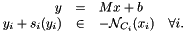where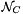denotes the normal cone to the set C. The dimensions of the sets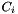should correspond to that of the blocks of rows of M. If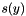is zero, we retrieve the optimality conditions of the quadratic minimization problem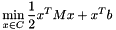Depending on the solver, M may need to be an explicit matrix (i.e. an instance of BlockMatrixBase), or simply an expression (for instance, a Product, NarySum, or a LinearSolverBase ).

The definition of the constraint set C and of the translation term s(y) is done by passing a NSLaw to the solve() function of the solvers. The NSLaw should conform to a specific interface, and provide at least

• NSLaw::eval(i,x_i,y_i) to evaluate the residual to the inclusion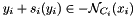at the i^th block
• NSLaw::dualityCOV(i, y_i, &s) to compute s_i(y_i)

Supplemental methods may need to be provided by the NSLaw depending on the solver chosen. See LCPLaw, SOCLaw or PyramidLaw for examples of the interfaces that should be provided by a NSLaw.

Implementations of Constrained Iterative Solvers include Projected Gauss Seidel (GaussSeidel, ProductGaussSeidel) and Projected Gradient (ProjectedGradient) , as well as the experimental ADMM and DualAMA classes.

# Projected Gauss Seidel

Constrained linear systems can be solved using the GaussSeidel or ProductGaussSeidel classes, as long as a NSLaw defining a corresponding NSLaw::solveLocal() function is available. SOCLaw, from the Second Order module, is an example of such NSlaw.

Example code for 3D Coulomb friction (requires the Second Order module):

//Construct the dual matrix
WType W = primal.H * ( primal.MInv * primal.H.transpose() ) ;
//Friction coefficients
const double * mu = { .... } ;
// Optional: connect our function to the callback, so we can monitor the GaussSeidel's convergence
gs.callback().connect( &ackCurrentResidual );
Eigen::VectorXd x( W.rows() ) ;
double res = gs.solve( bogus::Coulomb3D( n, mu ), b, x ) ;

System with supplemental linear equality constraints may be solved with the GaussSeidel::solveWithLinearConstraints function.

The ProductGaussSeidel class is useful when explicitely computing the matrix W would be expensive, but the product MInv * H' is quite sparse (that is, when updating a force component does not impact too many degrees of freedom). When Minv is the identity matrix, the above example can then be modified as

Eigen::VectorXd x( W.rows() ) ;
double res = gs.solve( bogus::Coulomb3D( n, mu ), b, x ) ;

When Minv is not the idenity matrix, one may use

bogus::ProductGaussSeidel< HType, MInvType > gs( primal.H, primal.MInv ) ;

The ProjectedGradient class may be used to compute the solution of the quadratic minimization problemusing a variant of the Projected Gradient or Projected Gradient Descent algorithms. Its interface is very similar to that of the above GaussSeidel. A few variants of the algorithm, such as the Nesterov  acceleration, are implemented; they can be selected using the ProjectedGradient::setDefaultVariant() method or using a template parameter. See projected_gradient::Variant for more information.

res = pg.solve( bogus::SOC3D( n, mu ), b, x ) ;
// ... or explicitely chose a variant
res = pg.solve< bogus::projected_gradient::APGD >( bogus::SOC3D( n, mu ), b, x ) ;

Once again, this algorithm can be used in a matrix-free fashion

typedef bogus::Product< JType, bogus::Transpose< JType > > Prod ; // Or use c++11 to infer the correct type
Prod W = J * J.transpose() ;
res = pg.solve( bogus::SOC3D( n, mu ), b, x ) ;

The NSLaw passed to the ProjectedGradient::solve() method should define a projectOnConstraint() function that computes the orthogonal projection onto the constraint set C.

Note
This algorithm should in theory only be used to solve constrained quadratic optimization problems. Coulomb friction does not belong to this class, but Linear and Cone Complementarity problems do. In practice, bogus does not disallow using a ProjectedGradient with a NSLaw for which the term s(y) is non-zero, but convergence may be degraded.